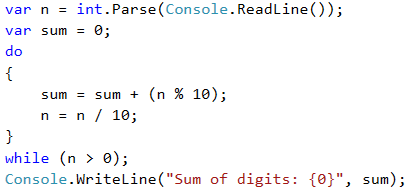Example: Summing Up Digits

Let's practice the do-while loop with the following exercise:

Sum up the digits of a positive integer n. Examples:

• If n = 5634, the result will be: 5 + 6 + 3 + 4 = 18.
• If n = 920, the result will be: 9 + 2 + 0 = 11.

Video: Sum of Digits

Watch this video lesson to learn how to sum the digits of given integer: https://youtu.be/sbzlzdoEbFc.

Hints and Guidelines

We can use the following idea to solve the problem: extract many times the last digit from the input number and sum the extracted digits until the input number reaches 0. Example:

• sum = 0
• n = 5634 🡒 extract 4; sum += 4; n = 563
• n = 563 🡒 extract 3; sum += 3; n = 56
• n = 56 🡒 extract 6; sum += 6; n = 5
• n = 5 🡒 extract 5; sum += 5; n = 0 🡒 end

In more detail the above idea looks like this:

• We create the variable n, to which we assign a value equal to the number entered by the user.
• We create a second variable – sum, which initial value is 0. We will use it for the calculation and storage of the result.
• As a loop condition, we will use n > 0 because after each calculation of the result in the body of the loop, we will remove the last digit of n.
• In the body of the loop:
• We assign a new value of sum that is the result of the sum of the current value of sum with the last digit of n.
• We assign a new value to n, which is the result of removing the last digit of n.
• Outside the body of the loop, we print the final value of the sum.

This is a sample code, implementing the above described steps:n % 10: returns the last digit of the number n. n / 10: deletes the last digit of n.